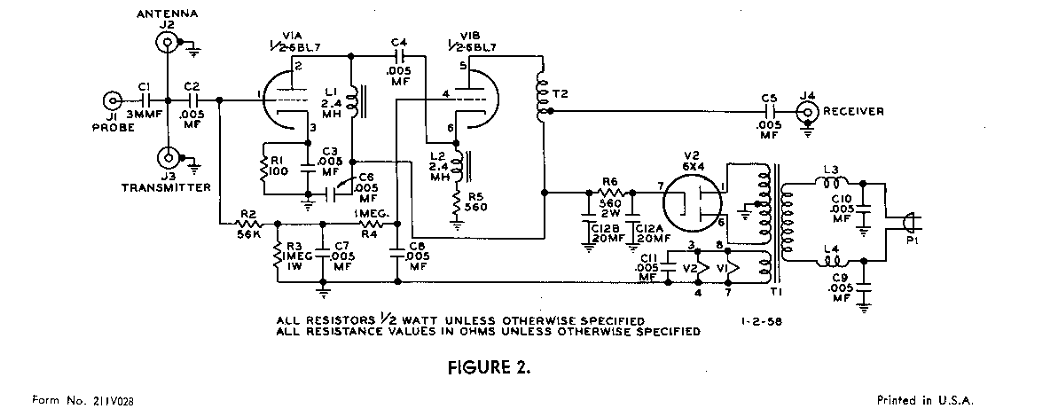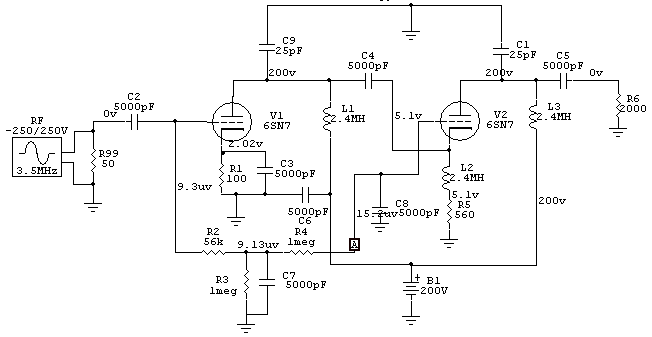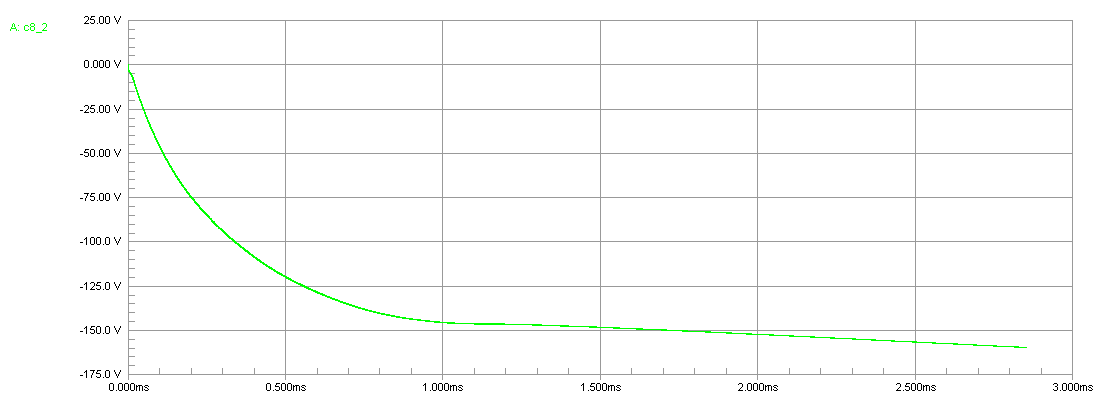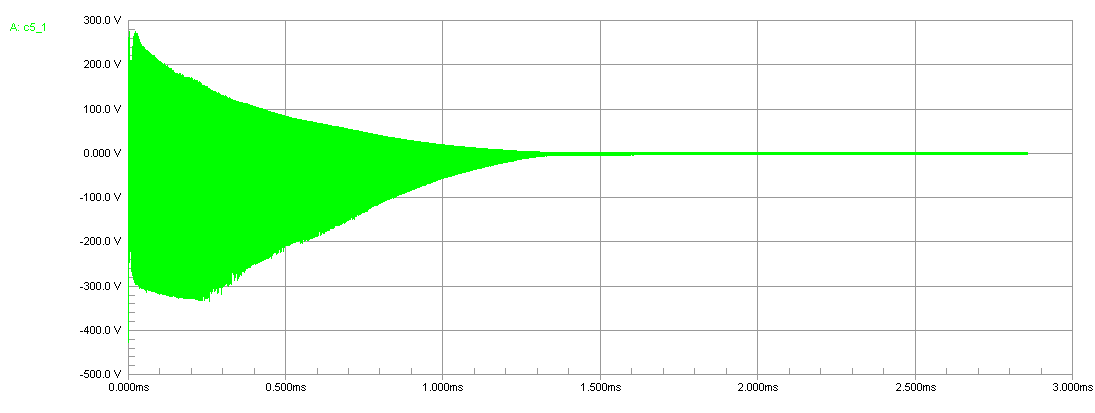Johnson TR Switch [ Home ] [ Up ]
 Ever wonder how a T/R switch works? Don't use it with a solid state receiver!Lets assume a 50 ohm load and 700 watts, which would have typically been maximum legal power back when this switch was made. The peak voltage at pin 1 of the 6BL7 on the first cycle would have been approximately 1.414 times the sqrt of 50*700, or 265 volts. Worse case when the transmission line has a zero impedance dc path, this voltage would charge C2 negative at pin 1, and positive towards J2 and J3. This would take several RF cycles. Grid current would, after C2 stabilizes at maximum charge level, be determined by grid leak R2. R2 limits charging current into C7 as the resistor-capacitor network R4 and C6 is charged negative. Maximum steady state grid current on pin 1 would be the current through R2 and R3, or about   177/1,056,000 = 160 uA. Peak current would be fairly high, dozens of mA, but also very brief. Peak current (assuming a square rise on the input envelope as worse case) would be the saturated grid-to-cathode current of the tube. It would be so brief that tube grid damage would be very unlikely. The grid would not have time to heat before C2 and C7 started to charge. After 1 or 2 milliseconds, the delay time set primarily by R4 and C8, pin 4 of the second section would go negative. Negative voltage would be roughly 150 volts, the same as the negative voltage at pin 1 caused by C2 charging negative on the pin 1 side. This further isolates the receiver, although it takes a brief time to do so.   My spice model uses a 6SN7, the closest low-mu triode to the 6BL7 I could find spice data for.Voltage at C8 (point A) below:RF voltage at R6 shown below:This voltage would be reduced by the step-down ratio of T2 in the actual switch. The actual voltage at the start would be less, with the T/R tube limiting most of the power. Peak power to the receiver would be several watts maximum for less than one millisecond. This most accurately shows the time before the tube cuts off.